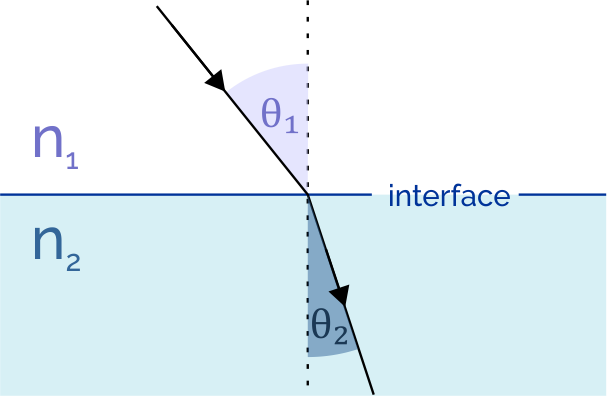# Snell's Law Calculator

Created by Bogna Szyk
Reviewed by Steven Wooding
Last updated: Dec 25, 2022

When light travels from one medium to another, it bends or refracts. The Snell's law calculator lets you explore this topic in detail and understand the principles of refraction. Read on to discover how Snell's law of refraction is formulated and what equation will let you calculate the angle of refraction. The last part of this article is devoted to the critical angle formula and definition.

## Snell's law of refraction

Snell's law describes how exactly refraction works. When a light ray enters a different medium, its speed and wavelength change. The ray bends either towards the normal of two media boundaries (when its speed decreases) or away from it (when its speed increases). The angle of refraction depends on the indices of refraction of both media:

$n_1 \sin(\theta_1) = n_2 \sin(\theta_2)$

where:

• $n_1$ is the refractive index of medium 1 (from which the ray travels);
• $n_2$ is the refractive index of medium 2 (into which the ray travels);
• $\theta_1$ is the angle of incidence - the angle between a line normal (perpendicular) to the boundary between two media and the incoming ray;
• $\theta_2$ is the angle of refraction - the angle between the normal to the boundary and the ray traveling through medium 2.

🔎 You can check how the speed of light can change in different media in the wave velocity calculator.

You can find some of the values of $n_1$ and $n_2$ for common media in the index of refraction calculator.Generally, Snell's law of refraction is only valid for isotropic media. In anisotropic ones, such as crystals, the ray may be split into two rays.

## Finding the angle of refraction - an example

Let's assume you want to find the angle of refraction of a light beam that travels from air to glass. The angle of incidence is 30°.

1. Find the index of refraction of air. It is equal to $1.000293$.
2. Find the index of refraction of glass. Let's assume it is equal to $1.50$.
3. Transform the equation so that the unknown (angle of refraction) is on the left-hand side: $\sin(\theta_2) = \frac{n_1 \sin(\theta_1)}{n_2}$.
4. Perform the calculations: $\sin(\theta_2) = \frac{1.000293 \sin(30\degree)}{1.50} = 0.333$.
5. Find the arcsin of this value: $\theta_2 = \arcsin(0.333) = 19.48 \degree$.
6. You can also save yourself some time and simply use the Snell's law calculator.

## Critical angle formula

Sometimes while applying the Snell's law of refraction, you will receive the $\sin(\theta_2)$ as a value greater than 1. This is, of course, impossible - our Snell's law calculator will display the value as "NaN". If this happens, it means that all light is reflected from the boundary (this phenomenon is known as the total internal reflection).

The highest angle of incidence, for which the light is not reflected, is called the critical angle. The refracted ray travels along the boundary between both media. It means that the angle of refraction is equal to 90°. Hence, you can find the critical angle by using the following equation:

$n_1 \sin(\theta_1) = n_2 \sin(\theta_2)$

After simplification, $n_1 \sin(\theta_1) = n_2 \cdot 1$.

Solving for the angle of incidence, $\theta_1 = \arcsin(\frac{n_2}{n_1})$.

🙋 Thirsty for more knowledge? Check our De Broglie wavelength calculator to read about the wave-particle duality, which explains light refraction.

Bogna SzykMedium 1
Vacuum
Refractive index 1 (n₁)
Medium 2
Air
Refractive index 2 (n₂)
Angle of incidence (θ₁)
deg
Angle of refraction (θ₂)
deg
People also viewed…

### BMR - Harris-Benedict equation

Harris-Benedict calculator uses one of the three most popular BMR formulas. Knowing your BMR (basal metabolic weight) may help you make important decisions about your diet and lifestyle.

### Car vs. Bike

Everyone knows biking is fantastic, but only this Car vs. Bike Calculator turns biking hours into trees! 🌳

### Diffraction grating

Diffraction grating calculator analyzes what happens when a light ray meets a surface with multiple apertures.

### Sled

Find out if a slope is safe to sled on with our sled calcultor!